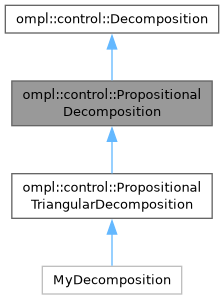ompl::control::PropositionalDecomposition Class Referenceabstract

A propositional decomposition wraps a given Decomposition with a region-to-proposition assignment operator. Each region in the decomposition has a corresponding World. More...

#include <ompl/control/planners/ltl/PropositionalDecomposition.h>

Inheritance diagram for ompl::control::PropositionalDecomposition:[legend]

## Public Member Functions

PropositionalDecomposition (const DecompositionPtr &decomp)
Creates a propositional decomposition wrapped around a given decomposition with a given number of propositions.

~PropositionalDecomposition () override
Clears all memory belonging to this propositional decomposition.

virtual World worldAtRegion (int rid)=0
Returns the World corresponding to a given region.

int getNumRegions () const override
Returns the number of regions in this propositional decomposition's underlying decomposition.

virtual int getNumProps () const =0
Returns the number of propositions in this propositional decomposition.

double getRegionVolume (int rid) override
Returns the volume of a given region.

int locateRegion (const base::State *s) const override
Returns the region of the underlying decomposition that contains a given State.

void project (const base::State *s, std::vector< double > &coord) const override
Project a given State to a set of coordinates in R^k, where k is the dimension of this Decomposition.

void getNeighbors (int rid, std::vector< int > &neighbors) const override
Stores a given region's neighbors into a given vector.

void sampleFromRegion (int rid, RNG &rng, std::vector< double > &coord) const override
Samples a projected coordinate from a given region.

void sampleFullState (const base::StateSamplerPtr &sampler, const std::vector< double > &coord, base::State *s) const override
Samples a State using a projected coordinate and a StateSampler.Public Member Functions inherited from ompl::control::Decomposition
Decomposition (int dim, const base::RealVectorBounds &b)
Constructor. Creates a Decomposition with a given dimension and a given set of bounds. Accepts as an optional argument a given number of regions.

virtual int getDimension () const
Returns the dimension of this Decomposition.

virtual const base::RealVectorBoundsgetBounds () const
Returns the bounds of this Decomposition.

## Protected Attributes

DecompositionPtr decomp_Protected Attributes inherited from ompl::control::Decomposition
int dimension_

base::RealVectorBounds bounds_

## Detailed Description

A propositional decomposition wraps a given Decomposition with a region-to-proposition assignment operator. Each region in the decomposition has a corresponding World.

Definition at line 127 of file PropositionalDecomposition.h.

The documentation for this class was generated from the following files: